+ +

# The path of the rays in the eye

## The path of light rays in the eye

This article is about the eye with respect to the laws of geometrical optics and its bright rays. We overlook for now the effects of diffraction, and consider that the rays can intersect in a point (rigorous stigma).  We have noted previously, that the retinal image is sharp, a basic prerequisite is that the rays emitted by the elementary point sources and picked up by the eye are focused in terms of the photosensitive retinal tissue. We have seen that when the rays were focused outside this plan, they formed on the retina a spot of light disc, more so wide that the rays were defocused.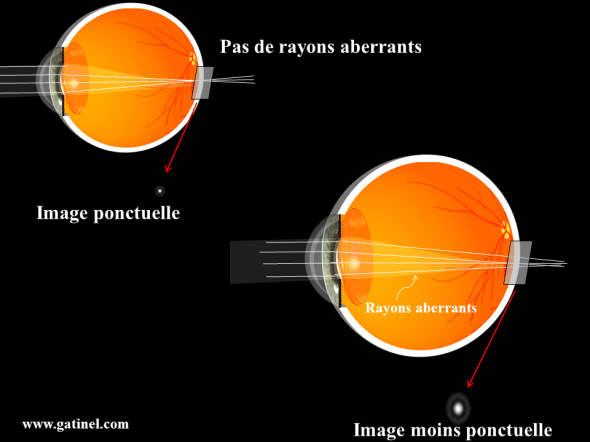When a part of the rays does not focus in the same plane as the majority of captured rays, the image quality is degraded. The image of a point is less punctual, and the contrast of the retinal image is reduced.

Envisage an intermediate situation: what's happening ' it when only a part of the rays picked up by the eye focuses in the retinal plan, and so that the other party focus outside? This issue is fully within the purview of the OPD, which aims to study comprehensively the journey of all rays captured by the pupil to deduce the PSF. The rays that do not converge in the plan of the retina are rays known as "aberrant" or "aberres". When all or some rays of light is not focused at the same point, we speak more generally of optical aberrations. It is one of the specificities of the OPD, which takes account of all the rays collected by the eye: who pass by or close to the center of the pupil, but also those who spend more at a distance, close to the edges, without forgetting those who are "in contact" of these edges (those are "scattered", it is actually more convenient in OPD to consider the incident as a "light wave front" light flow rather than a bunch of rays).

If a sufficient proportion captured rays intersect in the retinal plan, most of the details present in the observed pattern may be preserved within the retinal image, because the task of retinal illumination does not spread too... However, the contrast of the image will be affected.  The OPD can thus not only predict the Visual acuity of an eye, but also the way that improves the contrast of the retinal image. The OPD also allows to study situations where the eye a problem with optical lead specific retinal images, (ex: vision night halos, or ghosting splitting some luminous contours...).

It's time to report for the sake of simplicity, we talked of white, black, gray, etc. and as in the time of the beginnings of the cinema and television, overshadowed the polychromatic appearance of our surrounding world.  The principles outlined here also apply to different colored radiation, at the cost of small facilities of calculation that it is quite possible to ignore at this point. Just keep in mind that what is true for a 'red' RADIUS, is not always entirely accurate to a RADIUS 'blue '. For example, "blue" rays tend to form focal spots smaller that the rays 'red' (because as the blue radiation wavelength is smaller, they diffract less). That's why you can burn a disc "blu ray" in more detail (the disc is played with a "fine point of laser light blue') and that it contains more information than a DVD (read by a wider 'tip of red laser light').

By focusing on the characterization of the images and the color appearance of light, and features such as the wavelength, we have remote again shores of geometrical optics, that it is now time to return to permanently, to focus more closely on the way in which the captured light rays are heading in the eye. Thus, in everything that follows, we pursued the light information to a path of rays, skip the undulatory appearance of light, and stroll a world where the rays can intersect in a point: a world where the strong stigma is possible.

## The refraction of the eye

So that light rays (regardless of their 'own color') do not pursue the journey parallel or divergent, but 'start to converge"to the retina, it takes something to bend in the path, and make them"switch"in the direction of the Central retina. The human eye (like most mammals, and land and sea animals) has two remarkable fabrics in more ways than one: the cornea and the lens.

Through the cornea and the lens, the rays undergo deflections that are grouped together under the term of refraction: it is said that these rays are refracted. Many scholars became interested in the study of the laws that govern the path of light rays in the optical structures natural or artificial: the laws of refraction were established by Snell and Desmaps. The lens of the human eye is flexible, (at least prior to the introduction of presbyopia, to quarantine) and allows to the eye by deforming (cambering) to form a sharp image, even when highly divergent rays coming in contact with the eye. These rays are highly divergent, as issued by close sources - such as the pixels of the screen of your computer with your eyes). This mechanism is called accommodation of the lens.

When the eye (crystalline) is no longer able to deform enough that the rays converge sufficiently (accommodation of the lens becomes insufficient), needed a 'lens' external, i.e. a bezel convex glass, sort of "magnifying glass" that provides the extra convergence supplement. The use of these laws of refraction to understand how light rays are deflected, and serve to the development of more general formulas (many in geometric optics, but the most important is the so-called 'vergence' formula).

These formulas are designed in large part to answer a simple question: where the image forms after its refraction by the eye tissue? What interests especially the clinician, it is to know if these rays converge in the retinal plan... where outside. In addition to this legitimate curiosity, know the laws that govern the path of light rays also allows to calculate the optical power (the ability to do diverge the rays of light) of an artificial lens that once inserted in lieu of a clouded lens (cataract surgery).

Most commonly, these same formulas are useful to determine the curvature of a glass of glasses to correct nearsightedness, farsightedness, theastigmatism, or presbyopia. Finally, it may be interesting to know the size of the image on the retina, because of its relationship to the basic size of photosensitive elements, we can deduce the fineness of the details that will be "sampled", and those that will be lost... There are simple formulas for calculating the size of the images formed by an optical system. But before addressing these formulas over the pages of the site, it is certainly more important at this stage to understand the Genesis, the role and links.

## The law of refraction

We understood that the law of refraction is a pillar of geometrical optics, which flow formulas widely used to predict the path of light rays through optical media, and therefore the eye tissue. The law of refraction is deducted from the said principle of 'least action': it merely states that to go from one point to another, a ray of light will follow the shortest path in travel time. Here again, the light beam must be understood as a given abstract, which materialize the path of particles of light (photons), which would be issued in the direction of the ray. It is assumed that a photon emitted in a direction given in a homogeneous medium continues its path in a straight line (it is only upon detection of the photon that can deduce the route he took, because it is not possible to "follow" the path of a photon).

Let's take the example of a star light source. Of this star is born and spreads a signal light (or photon if one prefers to consider light as a stream of particles) which is transmitted in the surrounding space, at the speed of 300,000 Km seconds: to achieve what represents this speed, the light could have effected about 7 rounds of Earth since you have read the beginning of this sentence , or even made the journey to the moon. To do 7 laps of Earth per second, the light must travel in a vacuum (or air). In water, the time taken to complete the same course would be of about 1.33 times as long... Indeed, the light slows down in some quarters compared to the speed, unsurpassable, it adopts in a vacuum. Whatever the Middle crossing, light takes the time the shorter to go from one point to another: within a same light middle will therefore go straight to connect two points, because the straight line is the shortest distance between two points.

The fact that light systematically takes the shortest path temporally should not lead us to believe that would connect two points by a line to represent the direction of the ray. This method no longer works when the points are each located in two different backgrounds (ex: air and water). Indeed, we should not confuse duration and distance! At least, we can maintain this confusion until the Middle through the light does not change, and it is optically "homogeneous" (the starting point and the point of arrival are both located in the air or in water, or in the glass, and the light remains in the same medium during the entirety of his journey).

When light passes from one medium to another. the shortest route (in time) is more necessarily the shortest (by distance). We can guess the cause of this apparent paradox: it is that the speed of light varies according to the borrowed Middle. The light will never faster than in a vacuum, but his speed drops in some circles, "optically dense."

In the eye, for example, the speed of light is comparable to that which she would have in the water: it decreases is a third party about the study of the way of the light within the community requires to quantify precisely by means of a called number «» refractive index "(and designated by the letter"n") the way in which a material"brake"light (one speaks here of course not purely reflective and transparent materials that merely send the light, such as mirrors). The speed of light in a vacuum is used as reference.

If a medium to one index 1.5 (n = 1, 5), this means that the speed of light in a vacuum is 1.5 times greater than it takes in this environment. A direct consequence of this slowdown is that when the light has traveled 1.5 millimeter in a vacuum, she has only run only 1 millimeter in the middle. The product of the physical distance the refractive index is called the optical path.

To understand this concept, Let's imagine the trip made by a particle of light along a ray in an optical medium (n > 1) for a fixed period: as light slowed (n > 1), distance travelled is less than if the particle had journeyed into the void. The optical path expression corresponds to the physical distance that would have walked into the empty light during the same trip.

## Shortest path, or shortest path?

To predict the way in which light travels between two points located in two different environments, by crossing the interface between these environments, you can and should apply the principle of Fermat: light borrows the shortest (temporally) between two points. At this stage, it is important to understand that behind the simplicity of the principle of the shortest path, hide or even philosophical deeper questions: one could assume that the light emitted by the point located in the first environment could borrow any priori path to go to the point located in the second middle... or at least try all possible paths before you determine what is the shortest.

How does the RADIUS, where the particle of light which the Department materializes the direction of propagation, to know to start this shortest path? The answers to these questions are provided by the theory of quantum electrodynamics, and popularized by the physicist Richard Feynman. Regarding the quest for a law allowing to predict the path adopted by a refracted Ray, he must think of finalist way: using the principle of Fermat, who postulates that this will be the "fastest", the shorter in time, we must connect any point of a first middle (point n ° 1) (P1), with any point of a second environment (point n ° 2) (P2) by a single path , in minimum time.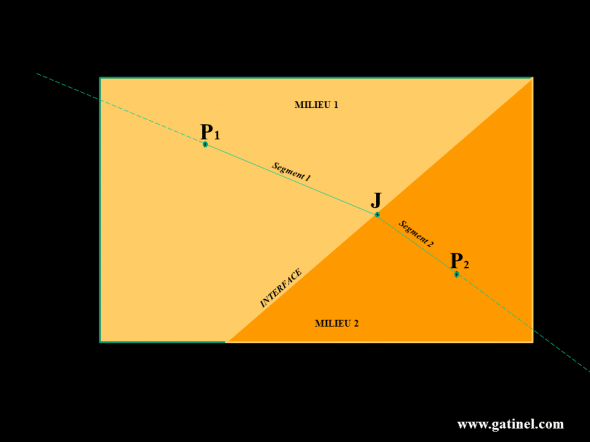The Middle 1 and the middle two are separated by a straight interface, but do not have the same index of refraction: the speed of light is not the same in the Middle than in the Middle 2 1. To go from the point 1, located in the Middle 1 to point 2, located in the middle of 2, there is a path of travel time minimum, consisting of two segments connected by a J point located at the junction (interface) of the circles. All RADIUS through the point 1 and directed according to segment 1 then borrows the path of segment 2 in the Middle 2. Whatever the point located on the right (P1J), the route will borrow a ray passing and directed by j. passes through the point n ° 2.

The ride will consist of two segments, since light travels in a straight line as long as it remains in a homogeneous medium. The first segment will go from point n ° 1 to a point located at junction of two circles (the point J, located along the interface), and the second segment will go from this point J to point No. 2.  As within the same medium, the minimum time to get from one point a the other is the straight line, we can deduce as a ray of light passing through the point n ° 1 and containing the first segment (and therefore by the J point) will continue its path in the second environment through the point n ° 2. Regardless of the position of the source at the origin of the ray in physiological optics, one seeks to determine the path of rays from a source within the eye circles, which we know geometry, refractive index... We are not truly trying to determine the shortest route between two points, but as we shall see. the resolution of this problem provides a general law (law of refraction) which allows to establish the route by a ray of light refracted within an interface.

An analogy with the "dilemma of the lifeguard" summarizes in a graphic way the problem of the shortest path.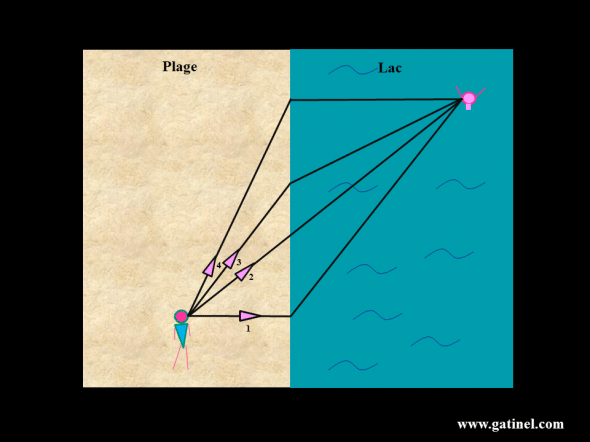To rescue the faster the bather in trouble, the lifeguard, who can't swim as fast (ex: 5 km/h) that he runs (ex: 15 km/h), must choose the "shortest" path (the one that requires the minimum travel time). If we look at the physical distance between the MN and the bather, path 2 is the shortest geometrically; It is looks shorter than the path 1 because of swimming distance is significantly longer than the distance race, and initially the 4 way swim distance is shorter, but the much longer race. However, the 3 way time could certainly be more brief, because even if the race is a bit longer, swimming is shorter and this provides a significant time saver! To solve the problem and find the course whose time is minimal, consider distances and speed of travel in covered areas (here the beach, and the water of the Lake). The time taken to cover a distance in a medium is equal to the ratio between the distance and the speed.
is called " optical path ("the distance in the Middle multiplied by the refractive index of the medium - index is an inverse function of the speed of light). The optical path in fact expresses a "temporal duration! On the basis of Fermat (the time traveled by the light between two points is the shortest), the equation of this situation development leads to the law of refraction known as Snell-Desmaps.

So suppose a lifeguard who sees a miserable rash swimmer about to drown from the banks of a lake. Knowing that to join the lifeguard will successively run and swim, and he swims faster that he runs, what is the "optimal" route, it will need to borrow? It is to this question, transposed to the field of "light rays", gave a clear answer Snell and Desmaps)more information on this law and its links with the propagation of light), at the end of series of painstaking observations and empirical montages. Sin (r) = (ni / nr) x sin (i)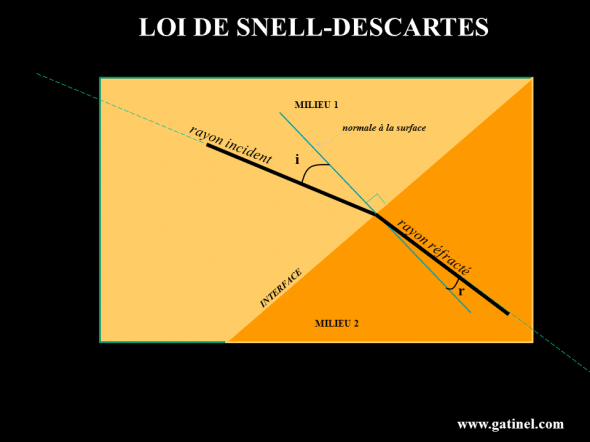The law of Snell Desmaps allows to know the angle of refraction, if one knows the direction of the incident Ray (its angle with the normal with the surface of the medium 2 to the point of 'contact'). The law of Snell Desmaps results from the generalization of the Fermat principle.

The sine of the angle of refraction is the ratio between the refractive indices multiplied by the sine of the angle of incidence. These angles are formed between the RADIUS and the line perpendicular to the interface at the contact point. This formula is a mathematical tool called "sinus", which is a ratio of two lengths, interconnected by an angle. For small angles, the sine of the angle is equal to the Angel himself. This law provides valuable information: only the angle of the incident with the surface radius and the report of the refractive indices determines the value of the angle of the refracted Ray. What counts, is the angle between the RADIUS with the interface and not the position of the source at the origin of the ray.  This Act involves logically the speed of light in circles considered, (expressed through the value of the indices of refraction).

Beyond the mathematical formalism, what the law teaches us, is that if one knows the movement speed of a ray of light in circles that it borrows, and incident management (the angle that make the rays when they meet the interface with the new medium), then We can calculate the angle under which it is deflected, IE "refracted".  Then, as long as the RADIUS remains in the same environment (same refractive index), are drive is carried out in a straight line, under the principle of economy as transport. If it encounters a new environment, simply determine the angle it forms with the interface between this one, and know its refractive index: can be applied again the law of Snell Desmaps. This approach, applied to RADIUS and RADIUS interface interface is called the "launch of rays" or «» ray tracing ». Tedious by hand, it has become common thanks to the computing done by computers where dedicated software allow to simulate the path of light in optical models.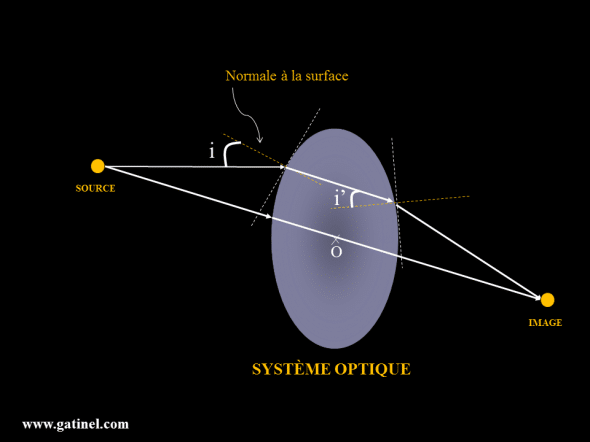The laws of refraction allow to trace the path of the rays through an optical system which we know geometry (he must know their local curvature, to position normal to the surface, to determine the angle of incidence, and calculate the angle of refraction through the law of refraction). This can be useful to determine the position of the plant of the sharp image of a source object: we draw several rays, and we see where they intersect. You can also use some simplified forms (or the fact that the rays which pass through the center of the optical system are not deflected), which are used to determine the position where the rays emitted by a source which we knows the distance with the optical system will focus (position of the image).

Ray tracing is used to develop optical solutions, ranging from eyeglasses to eye lenses, implants, etc. We model the eye and the solution tested, and calculating with a computer the path of rays when they spread within the eye and tested equipment. These simulations are used to define an optimal geometry of optical equipment. When looking to simply predict the position of an image formed by the eye (is it in terms of the retina?), and that is not a ray tracing software used, you can also use a called approximation formula of vergencewhich stems from the law of Snell Desmaps. Vergence formula does not require that we calculate the path of rays Special: it simply appeals to the position of the object at the optical system, and the distance at which this system focuses the rays (the inverse of the focal distance is called vergence).

The formula of vergence is an efficient and simple way to calculate the position of an image after refraction of rays from the top of a lens or a diopter (IE plan where if we put a screen, the image formed would be the sharper because it is equal to the intersection of the refracted rays plan).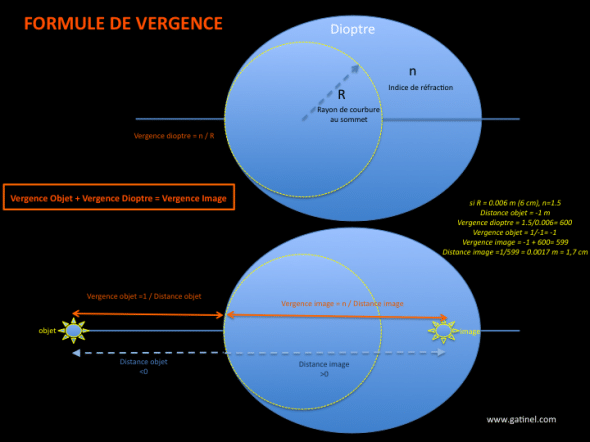A diopter placed in air (n = 1) has a refractive index and an apical curvature (at the top, along the optical axis) that are used to calculate its vergence. If one knows the position of the object at the top of the diopter, can then be calculated the position of the image through the vergence formula, which States that the sum of the vergence of the (inverse of the distance in the air) object added to the vergence of the dioptre is equal to the distance of the image. By convention, the distances "to the right" are positive sign.

It is therefore the principle of Fermat, any action that follows the most taught law of geometrical optics, refraction law, or law of Sines. If the principle of Fermat is the keystone of the optical theories that endeavour to describe the path of light in classic conditions; the law of Sines forms the main pillar of the scaffolding of the theoretical formulas used commonly in geometrical optics, as the formula of vergence.